# Introduction - Towards Discoverability

Results are most impactful when they are reproducible, understandable, and discoverable; Analysts cannot incorporate a finding into their understanding of a particular dataset or question unless they know that result exists. This can be difficult within the status quo, where results are often sent directly to collaborators on a particular project, and then (hopefully) archived - often in a location and manner specific to the analyst who generated them.

Results are discoverable on the other hand, when there is a reasonable mechanism by which anyone with appropriate access permissions can discover the existance of - and locate - them. The searching party might be a new collaborator getting up to speed on a project, a scientist working in the same space and trying to determine what has already been done, or even the analyst themselves looking for a result generated months or years previous.

The trackr package seeks to improve the discoverability of results by both recording the existence of (and in some cases object representing) R-based results in a customizable database, and annotating those rescords with automatically inferred metadata about those results. These annotations power the ability to search for and find records of particular results, or classes thereof, whether or not the seeker knew of them beforehand.

# Dependency installation check

To run all examples and vignettes, the following packages should be installed:

• From CRAN: ggplot2, lattice, proto, digest, png, httr, MASS, MEMSS, mlmRev, devtools, CodeDepends, rsolr
• From Bioconductor: BiocGenerics

# Setup

## Use temporary trackr backend

By default trackr will write to a permanent default JSON backend which lives at ~/.trackr/objdb.json. For the purposes of this vignette, we point it at a temporary one so the vignette does not create permanent files as it is running.

Users will generally not need to run the code below, though they may choose to utilize a non-default backend in which case they will need to specify that in their session.

library(trackr)

tdb = TrackrDB(backend = JSONBackend(file = file.path(tempdir(), "objdb.json")),
img_dir = file.path(tempdir(), "trackr_img_dir"))

defaultTDB(tdb)
## An object of class "TrackrDB"
## Slot "opts":
## An object of class "TrackrOptions"
## Slot "insert_delay":
##  0
##
## Slot "img_dir":
##  "/tmp/RtmpMWHMja/trackr_img_dir"
##
## Slot "img_ext":
##  "png"
##
## Slot "backend_opts":
## list()
##
##
## Slot "backend":
## Reference class object of class "JSONBackend"
## Field "docs":
## DocList (0x0)
## Field ".file":
##  "/tmp/RtmpMWHMja/objdb.json"
## Field "file":
##  "/tmp/RtmpMWHMja/objdb.json"

## Create some example plots

Here we create a number of plots - ggplot2, lattice, and base - which we will use throughout the demonstration. The details of these plots themselves is not important, but we use many different datasets and plotting methodologies in order to illustrate the different avenues for discoverability enabled by trackr’s automatic annotations.

library(ggplot2)
library(lattice)

## examples from
##      http://www.cookbook-r.com/Graphs
##      https://learnr.files.wordpress.com/2009/08/latbook.pdf
##      http://docs.ggplot2.org/current/index.html
##      http://lmdvr.r-forge.r-project.org/figures/figures.html

## modified version of Figure 1.1 in
## http://lmdvr.r-forge.r-project.org/figures/figures.html
data(Chem97, package = "mlmRev")
pl <- histogram(~gcsescore | factor(score),
data = Chem97, main="Lattice Histogram of gcsescore", sub="conditioned on score")
pl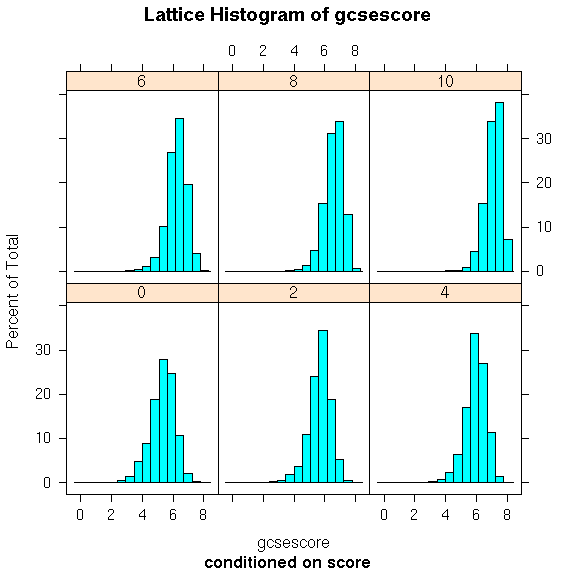## and a modified version of its ggplot2 counterpart, from
## https://learnr.files.wordpress.com/2009/08/latbook.pdf
pg <- ggplot(Chem97, aes(gcsescore)) +
geom_histogram(binwidth = 0.5) +
facet_wrap(~score) +
ggtitle(expression(atop("ggplot2 Histogram of gcsescore", atop("facetted by score")))) +
theme_bw()
pg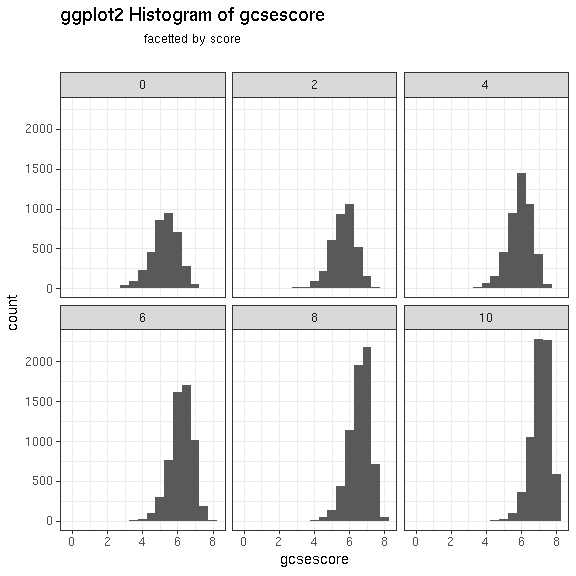## modified version of a graphic available here:
## http://docs.ggplot2.org/current/scale_hue.html
set.seed(620)
dsamp <- diamonds[sample(nrow(diamonds), 1000), ]
d <- ggplot(dsamp, aes(carat, price, colour = clarity)) +
ggtitle("Diamond price by carat and clarity") +
geom_point() + theme_bw()
d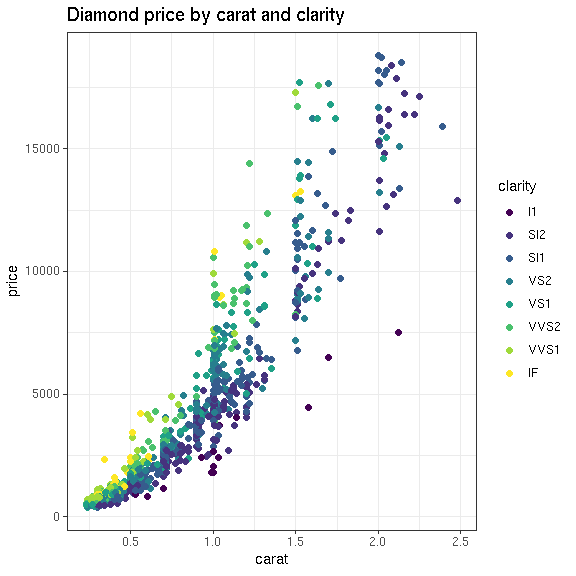## modified version of a graphic available here:
## http://docs.ggplot2.org/current/stat_density2d.html
data(geyser, package = "MASS")
m <- ggplot(geyser, aes(x = duration, y = waiting)) +
geom_point() + geom_density2d() +
xlim(0.5, 6) + ylim(40, 110) +
xlab("Eruption time in min") + ylab("Waiting time in min") +
ggtitle("Eruption length and waiting time") +
coord_trans(y="log10") + theme_bw()
m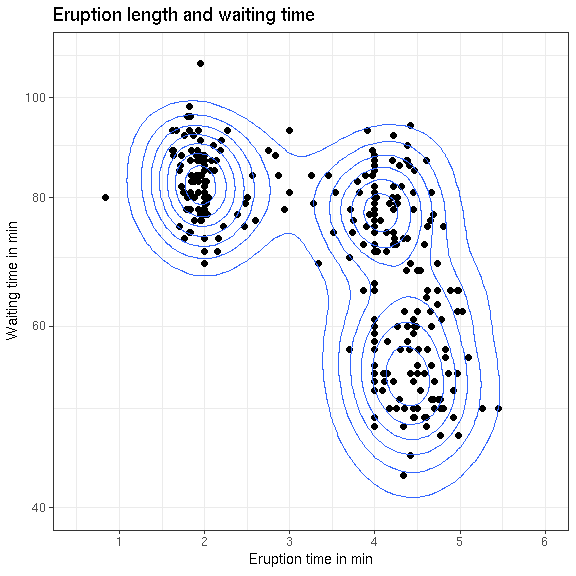## modified version of Figure 2.1 in
## http://lmdvr.r-forge.r-project.org/figures/figures.html
data(Oats, package = "MEMSS")
tp1.oats <- xyplot(yield ~ nitro | Variety + Block, data = Oats,
type = 'o',
xlab = "Nitrogen conc (cwt/acre)",
ylab = "Yield (bushels/acre)",
main = "Yield by nitrogen | variety + block")
tp1.oats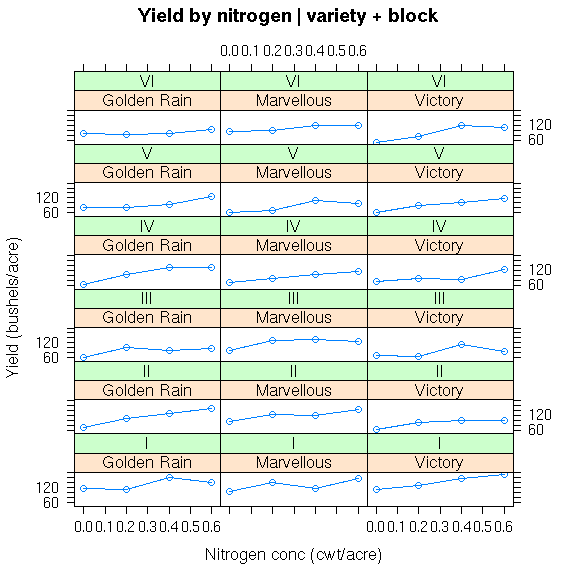## and a modified version of its ggplot2 counterpart, from
## https://learnr.files.wordpress.com/2009/08/latbook.pdf
pg.oats <- ggplot(Oats, aes(nitro, yield)) +
geom_line() + geom_point(shape = 'o') +
facet_grid(Block ~ Variety) +
xlab("Nitrogen concentration (cwt/acre)") +
ylab("Yield (bushels/acre)") +
ggtitle("Yeild by nitrogen, facetted by variety and block") + theme_bw()
pg.oats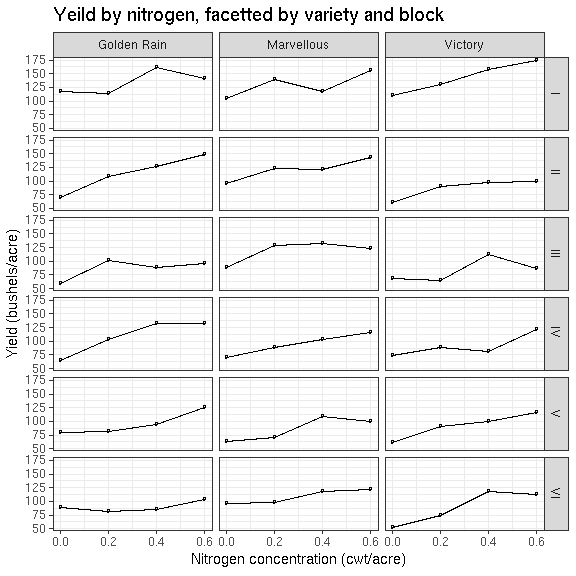## Figure 1.2 in
## http://lmdvr.r-forge.r-project.org/figures/figures.html
pl2 <- densityplot(~ gcsescore | factor(score), data = Chem97,
plot.points = FALSE, ref = TRUE, main = "Density of gcsescore", subtitle= "conditioned on score")
pl2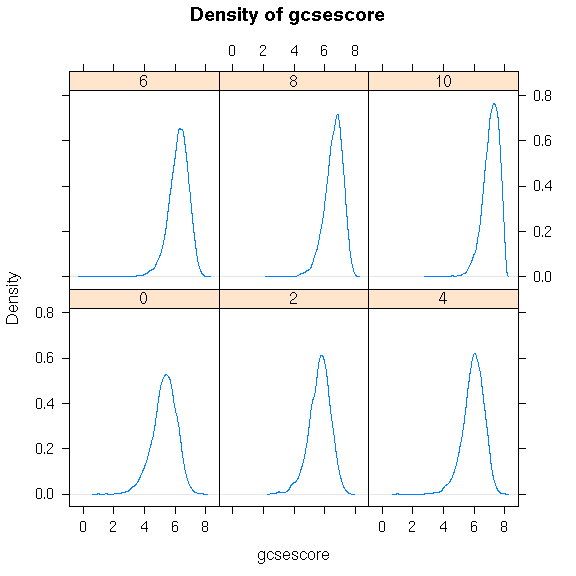## and a modified version of its ggplot2 counterpart, from
## https://learnr.files.wordpress.com/2009/08/latbook.pdf
pg2 <- ggplot(Chem97, aes(gcsescore)) +
stat_density(geom = "path", position = "identity") +
facet_wrap(~score) +
ggtitle(expression(atop("Density of gcsescore", atop(" facetted by score")))) + theme_bw()
pg2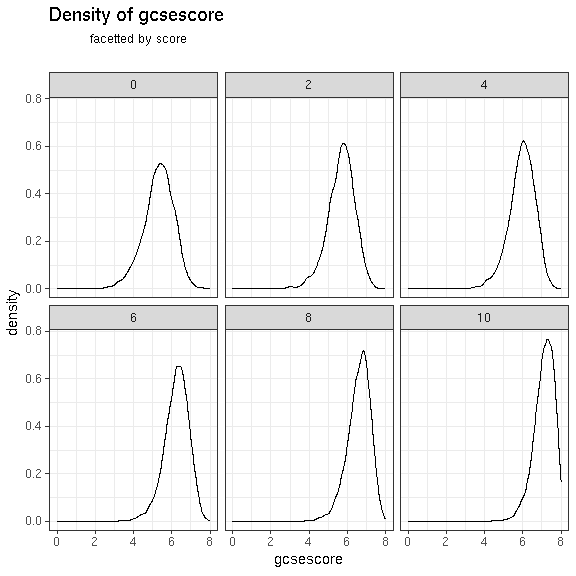## a modified version of a graphic available here:
## http://docs.ggplot2.org/current/coord_trans.html
df.abc <- data.frame(a = abs(rnorm(26)), letters)
pg3 <- ggplot(df.abc, aes(a, letters)) +
geom_point() + coord_trans(x = "sqrt") +
annotate("text", x = 1.25, y = 5, label = "Some text") +
ggtitle("Simulated normal data vs letters") +
theme_bw()
pg3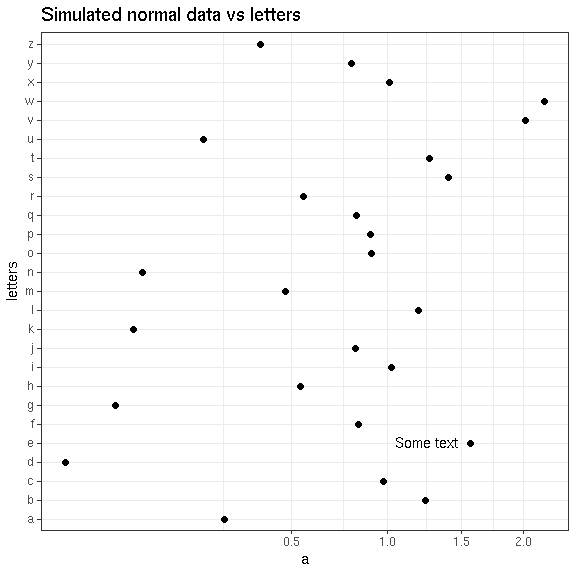## and a lattice version to match
pl3 <- xyplot(letters ~ a, data = df.abc,
panel = function(x, y,...) {
panel.xyplot(x, y,...)
panel.text(1.25, 5, labels = "Some text")
},
main = "Simulated data vs letters v2"
)
pl3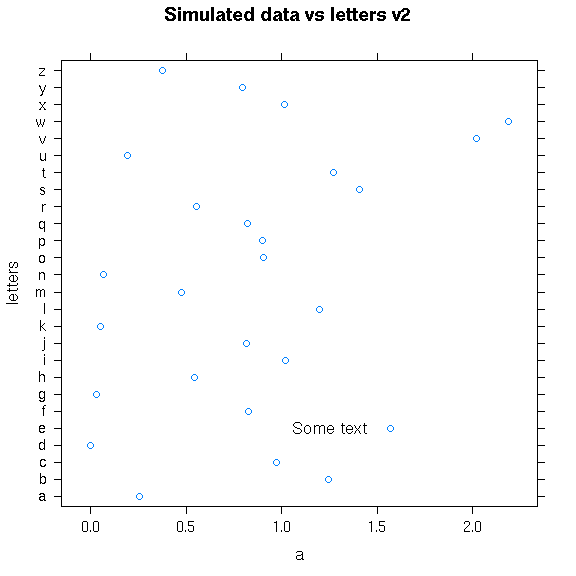## Figure 1.3 in
## http://lmdvr.r-forge.r-project.org/figures/figures.html
pl4 <- densityplot(~ gcsescore, data = Chem97, groups = score,
plot.points = FALSE, ref = TRUE,
auto.key = list(columns = 3),
main = "Densities of gcsescore by score")
pl4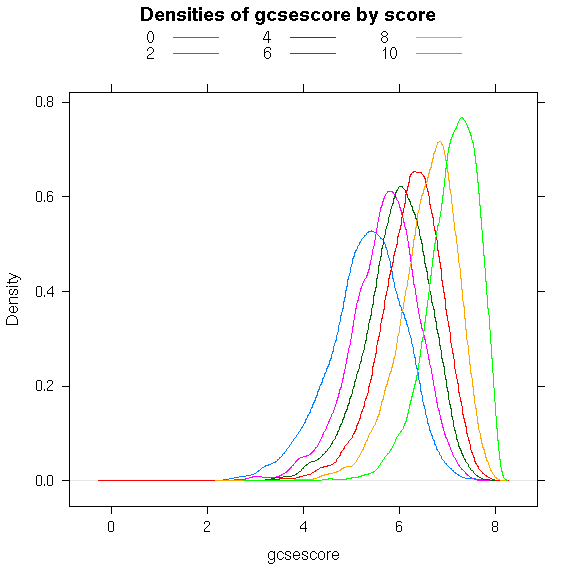## and a modified version of its ggplot2 counterpart, from
## https://learnr.files.wordpress.com/2009/08/latbook.pdf
pg4 <- ggplot(Chem97, aes(gcsescore)) +
stat_density(geom = "path", position = "identity",
aes(colour = factor(score))) +
ggtitle("Densities of gcsescore by score 2") + theme_bw() +
theme(legend.position = 'top')
pg4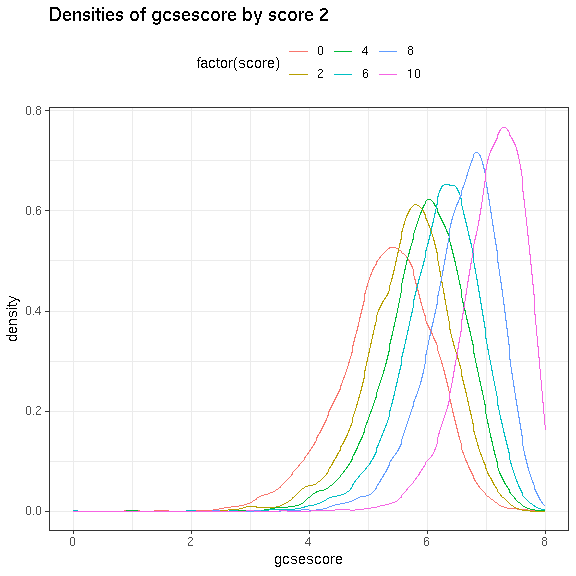## a custom example, with both lattice and ggplot2 versions
x = sample(1:5, 1000, replace=TRUE)
y = rnorm(1000, x, sd = 1/sqrt(x))
lp = densityplot(~y, groups = x,
auto.key=list(space="right", title="x", points=TRUE), main = "More simulated data")
lp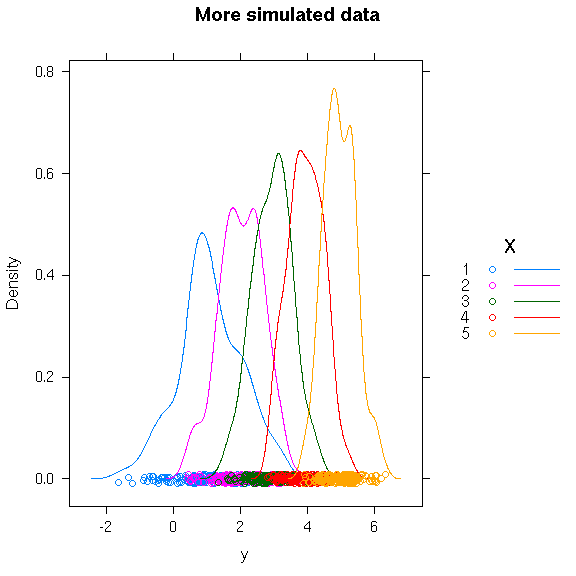gp = ggplot(data.frame(x = x, y = y),
aes(x = y, colour = as.factor(x))) +
stat_density(geom = "path", position = "identity") +
geom_point(aes(y = 0),
position = position_jitter(height = 0.01), shape = 21) +
ggtitle("More simulated data v2") +
theme_bw()
gp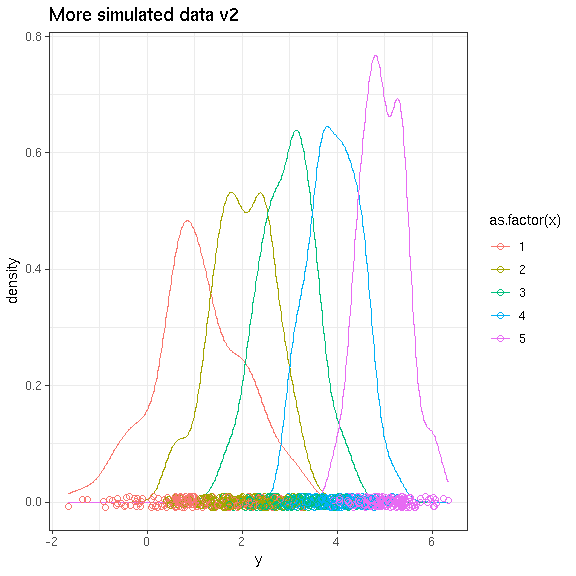## and a couple of base graphics plots
## note that these must be assigned to a variable using recordPlot()
plot(1:10, 1:10, main = "1:10 by 1:10")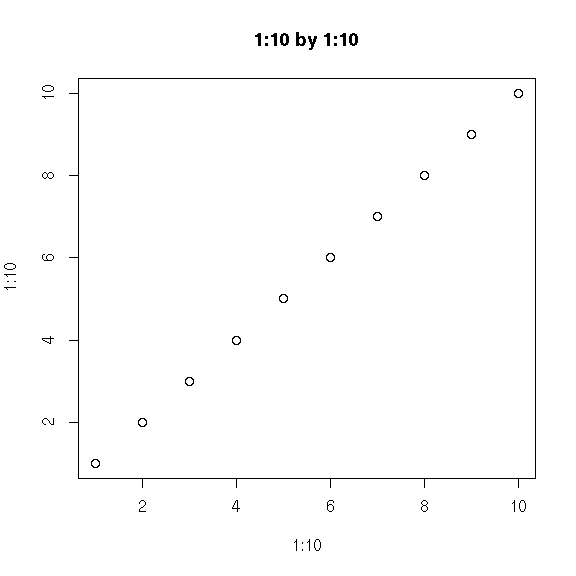pb1 <- recordPlot()

par(mfrow = c(2, 3))
par(cex = 0.6)
par(mar = c(3, 3, 0, 0), oma = c(1, 1, 1, 1))
for (i in 1:6) {
plot(1, 1, type = "n")
mtext(letters[i], side = 3, line = -1, adj = 0.1, cex = 0.6)
}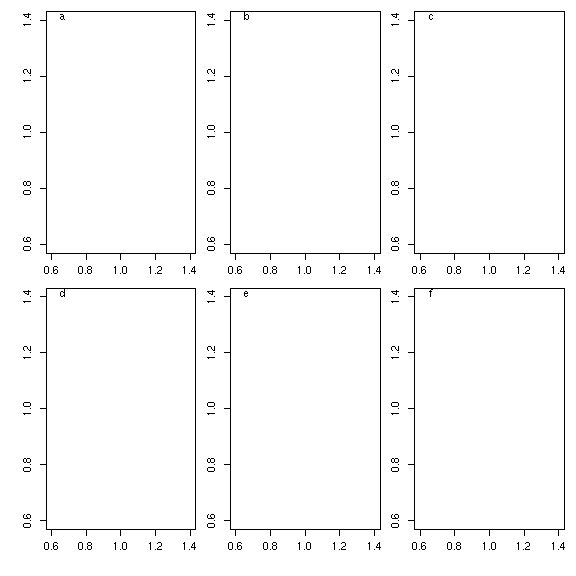pb2 <- recordPlot()

# Recording results with trackr

Recording a result adds a record of it - annotated with the inferred metadata - to the database of results in the active trackr backend. Whether the result itself is cached in a serialized form as part of the recording process depends on the backend in use.

## R objects and visualizations

To record an object (plot or otherwise) in our R session with trackr we use the record() function. Generally we can simply call record with the only argument being the object/result to record, like so:

record(pl)
## Warning in FUN(X[[i]], ...): input variable lettersnot found as previous
## output.Looking for current value in .GlobalEnv.
## Warning in FUN(X[[i]], ...): input variable scorenot found as previous
## output.Looking for current value in .GlobalEnv.
## Error in FUN(X[[i]], ...): Unable to determine hash and class of input var score

When calling record from within e.g., an apply-style call, however, we need to specify the symbol (or position) representing the object in the sequence of top-level expressions captured by histry via symorpos arugment. We will do this while submitting the remaining plots as a list. With a few particular exceptions, it is not recommended to call record from within a function body.

Generally results will be recorded one-at-a-time in the manner described initially. We do it via mapply here soley here for our convenience as the authors of this vignette.

my.plots <- list(pg = pg,
d = d,
m = m,
tp1.oats = tp1.oats,
pg.oats = pg.oats,
pl2 = pl2,
pg2 = pg2,
pg3 = pg3,
pl3 = pl3,
pl4 = pl4,
pg4 = pg4,
lp = lp,
gp = gp,
pb1 = pb1,
pb2 = pb2)
invisible(mapply(record, object = my.plots, symorpos = names(my.plots)))
## Warning in FUN(X[[i]], ...): input variable lettersnot found as previous
## output.Looking for current value in .GlobalEnv.
## Warning in FUN(X[[i]], ...): input variable scorenot found as previous
## output.Looking for current value in .GlobalEnv.
## Error in FUN(X[[i]], ...): Unable to determine hash and class of input var score

## Rmd/Rnw reports

Trackr also supports recording Dynamic reports generated via knitr/rmarkdown. To do this we would call the knit_and_record function. The function takes a dynamic report source document (e.g., an Rmd or Rnw file), any arguments that can be passed to rmarkdown’s render() function, and some further arguments which generally won’t need to be set by the user.

We do not run the code below within this vignette due to already being in a knitr context.

# Not run
knit_and_record("fancyreport.Rmd")

# Finding results vai the trackr R API

Now that our results have been recorded by trackr, we can discover them. trackr includes a search function called findRecords(), which allows us to search the active backend for records of results that match various criteria.

By default findRecords returns a DocList object (defined in the rsolr package). While this is a ragged data structure, we can treat it as if it is a sparse tabular data.frame and select “columns” out of it. We do this for the titles field below.

By searching for "density", we can see that 7 of our 16 plots are associated with that term, either by their title, having been generated with the lattice-based densityPlot function, or involving either geom_density or stat_density2d from ggplot2.

res = findRecords("density")
res[,"titles"]
## list()

We can also find plots based on their title. The exact search syntax depends somewhat on the backend. Our JSON backend accepts regular expressions, whilea Solr-based backend would accept syntax specific to that system.

findRecords("[sS]imulated")[,"titles"]
## list()

We get the same result when we restrict our search to matching the titles field specifically via the fields argument:

findRecords("[sS]imulated", fields="titles")[,"titles"]
## list()

The names of variables and datasets are often useful to search for. This can allow us to find multiple plots which appear to be related to eachother (by the fact that they visualize different aspects of the same dataset).

findRecords("Oats")[,"titles"]
## list()

Note that this is true even when a modified version of the dataset is what is actually plotted (only a sample of the diamonds dataset, stored in a separate dsamp variable, was used in the diamonds plot)

diamres = findRecords("diamonds")
diamres[,"titles"]
## list()

The records created by trackr include the code used to generate the plot, which we can easily look at and evaluate:

rm(d)
print(diamres[]$code) ## Error in diamres[]: subscript out of bounds eval(parse(text = diamres[]$code))
## Error in diamres[]: subscript out of bounds
d
## Error in eval(expr, envir, enclos): object 'd' not found

This allows us to treat code that generated previous results as “Code snippets” or recipes to generate a particular type of result.

We can also search for plots where a paritcular variable is used. This might give return many different types of plots where that variable was used, allowing us to get a more hollistic approach to how analysts have approached that variable. In the code below we dot his by evaluating the code to get each plot object, and using the gridExtra package to arrange

gcseplots = findRecords("gcsescore", fields = "code")
library(gridExtra)
plotobjs = lapply(seq(along=gcseplots), function(i) {
cd = gcseplots[[i]]$code plt = eval(parse(text = cd)) plt }) gallery = marrangeGrob(plotobjs, nrow =2, ncol=3) gallery We can see above that in our set of example plots, gcsescore is is looked at primarily in terms of its distribution conditional on a particular value for score. ## Recreating the package cohort for a result With the suggested switchr package installed, we can create a SessionManifest from a record (as returned by findRecords) by calling the manifestFromRecord function: library(switchr) man = manifestFromRecord(diamres[]) ## Error in is(lst, "list"): subscript out of bounds man ## Error in eval(expr, envir, enclos): object 'man' not found We could then use this manifest to recreate the environment (up to R package versions) used to create the result (NOT RUN) nm = gsub(":", "_", diamres[]$id)
switchTo(nm, seed = man)

## OR

install_packages(man)

# Integrating with Blacklight via rsolr

Note that the following examples assume that a solr instance is installed as a part of a blacklight instance (at ~/Downloads/trackr_blacklightapp), is properly configured and is running/awaiting input with a core named figdb.

## NOT RUN

library(rsolr)

blcklght.img.dir <- "~/Downloads/trackr_blacklightapp/app/assets/images"
solr.uri <- "http://localhost:8983/solr/figdb"
## non-standard requestHandler not strictly required,
## but will maintain consistency with search results received via UI
sl <- SolrList(solr.uri, requestHandler="search")
tdb = TrackrDB(TrackrOptions(img_dir = blklght.img.dir), backend = sl)
invisible(lapply(my.pfs, record, sl, db = tdb))

regDateTime(my.pfs[]) <- Sys.time() - as.difftime(30, unit = "days")
## note that this does not change the unique ID
## (unique ID determined by hash of plot object at creation time of original PlotFeatureSet)
## and so the document is overwritten in the data store.
record(my.pfs[], db = tdb, verbose=TRUE)

my.pfs[] <- editTags(my.pfs[], c("hello", "world", "goodbye"), option="add")
## same as above (document is overwritten)
record(my.pfs[], db = tdb, verbose=TRUE)
res <- findRecords("density", tdb)
ndoc(res)
res[1,"image.path"]
loadEnrichedPlot(file.path(blcklght.img.dir, res[1,"image.path"]))

res <- rmRecord(uniqueID(my.pfs[]),db = tdb)
ndoc(res)
rmRecord(my.pfs[], db = tdb, verbose=TRUE)
res <- findRecords(uniqueID(my.pfs[]), db = tdb, fields="id")
ndoc(res)
record(my.pfs[], db = tdb, verbose=TRUE)